### POSTER SESSION 2

EFFECTS OF THE RADIATIVE TRANSFER OF THE VAPOR PHASE ON THE BOIL-OFF RATES

A. BENBRIK, M. RIGHI, A ELAHOUEL
National Institut of Hydrocarbons, Boumerdes, ALGERIA.

ABSTRACT

Algeria is one of the greatest producing countries of natural gas . For its export to consumer countries it is liquified and stored at a temperature of -162oC in a cryogenic storage tanks of a capacity of 56000 m3, and a pressure slightly greater than atmospheric pressure. Thermal insulation of these tanks is realized by the perlite or the fiberglass. Despite that, the infiltration of the middle ambient heat to the inner of the tank remains always present. Consequently the natural liquified gas evaporates. These vapors are called Boil-off. The maximum boil-off designed is 0.08 % of the total storage tank volume per day. The stored LNG is a mixture of Methane, and heavy Hydrocarbons with a weak percentage of nitrogen, these vapors are mainly constiuted of Methane. The Boil-off represents a sensitive loss. And also affects the physical and chemical properties of the LNG. Cryogenic storage tanks are never completely flled. Enough vapor space is required to provide adequate volume boiling. The minimum vapor space is about 1/2% of the total storage volume. The heat transfer into the portion of the tank above the liquid level is controlled by convection and radiant cooling on the inside surface; this vapor space heat leak cannot be neglected, or the calculated Boil-off rate will be substantially low. The volume of the vapor varies accordingly to the liquid level inside the tank. This vapor which is methane is considered as a semi transparent media.

Our aim in this work is to study the influence of the transfer heat by thermal radiation of the semi transparent media on the boil-off rate. The space of the vapor phase is limited by the roof of the Tank, the cylindrical wall of the liner , and the surface of the liquid surface of LGN. Adjacent surface temperatures are known, as well as their emissivity coefficients. The surface of the liquid is considered black.

In this study we have to solve this problem of radiation effect in the semi transparent media by using Discrete Ordinates Method. Specific cases of different storage parameters such as liquid level and vapor pressure are taken into account.

Obtained results have confirmed the influence of the thermal radiation on the boil-off rate.

REFERENCES

1. Adorjan, A.S., Heat Transfer in LNG Engineering, Hemisphere Publishing Corpration,1991.
2. Neil, D., Hashemi, H.T. and Sliepcevich, C.M., Boil-off and Wall Temperatures in Aboveground LNG Storage Tanks, Advances in Cryogenic Heat Transfer, Chemical Engineering Progress Symposium Series.A THEORETICAL AND EXPERIMENTAL INVESTIGATION OF A RADIATIVE COOLING SYSTEM

Moh'd A. Al-Nimr, Z. Kodah and B.E. Nassar
Mechanical Engineering Department.
J. U. S. T.
Irbid - Jordan

ABSTRACT

A radiative cooling system is built in Irbid-Jordan and utilized to cool and store fluids by direct radiation to the low effective sky temperature. The radiative cooling system is built to verify the validity of a mathematical model proposed to describe the system dynamic thermal behaviour. The constructed, radiative system has the ability to create a 15 oC reduction in the mean temperature of a 120 liter capacity storage tank over one night, this implies that the system can emit 13 MJ / (m2. night) to the sky. It is found that the theoretical and the experimental predictions of the proposed model are in good agreement.

EXPERIMENTAL STUDY OF RADIATIVE PROPERTIES OF PARTICLES DURING COAL CONVERSION PROCESS

Anatoly P. NEFEDOV, Olga S. VAULINA, Oleg F. PETROV,. Alex A. SAMARIAN
High Energy Density Research Center,
Russian Academy of Sciences, IVTAN, Moscow, Russia

ABSTRACT

The work described here represents our studies of radiative properties of pulverized coal particles during conversion process. Experiments on a coal- fired Meeker burner are detailed. To conduct experiments the novel optical diagnostic techniques have been used which allowed to measure temperatures of particles and gas; mean sizes, refractive index and concentration of particles; optical density, and spectral emissivity of a cloud of coal parricles. The spectral emissivity and single scattering albedo measurements have been carried out at the different step of the conversion process of coal particles. These measurements allowed to make a conclusion about the contribution of the particle emittance into radiative transfer in the flow of the combustion products.

APPLICATION OF THE BOUNDARY ELEMENT METHOD IN RADIATION

Ryszard A. Bialecki, Lukasz Grela*
Institute of Thermal Technology, Silesian Technical University, Gliwice, Poland1
*Power Plant Laziska, Poland

1. INTRODUCTION

The paper shows two new applications of the Boundary Element Method (BEM) in heat radiation: solving radiation transfer problems in emitting absorbing media and solving heat transfer problems in solids containing self irradiating portions of the boundaries.

BEM is a numerical technique used to solve boundary value problems1. The field of application of this method is similar to the field methods as FEM and FDM. While in the field methods the original differential equations are being discretized, the objects discretized in BEM are integral equations. The latter are obtained by applying the reciprocity theorem to the boundary value problem at hand. As a discretization technique of integral equations BEM is an efficient tool to handle integral equations of heat radiation. Moreover, because the singular behaviour of the kernels arising in equations of radiation and that of standard BEM are the same2, the application of BEM in radiation is straightforward.

2. HEAT RADIATION IN PARTICIPATING MEDIA

Hottel's zoning method is a well established tool of solving radiative heat transfer problems. Several improvements of the classic zoning technique have been reported in the literature3. It has been shown recently2, that the original Hottel's formulation can be interpreted as a Galerkin discretization of the integral equations of heat radiation.

From the standpoint of the numerical implementation, the main drawback of he zoning technique is its long computer running time. Other inherent difficulty of this approach is the poor accuracy of numerical evaluation of matrix entries (exchange areas). Geometries that stray form purely rectangular or cylindrical are difficult to handle by zoning techniques. Boundary Element Method (BEM) offers simple way to bypass all these difficulties.

Consider a volume V filled with participating medium and bounded by a surface S being a diffuse emitter and reflector of radiation. For such a configuration the governing equations of heat radiation link four functions: blackbody emissive power eb[T] of the surface S, blackbody emissive power of the medium eb[Tm] filling the domain, radiative heat flux qr on the bounding surface, and radiative heat source qvr within the domain. Energy balance on an differential surface element yields the first governing integral equation2(1)

where: b- radiosity,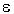- emissivity, a- absorption coefficient,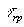- transmissivity between current point r and observation point p, K, K1 - kernel functions proportional to |r-p|-2. Surface and volume integrals arising on the right hand side of Eq. 1 will be denoted as I and Iv, respectively. Similar balance written for differential volume of the medium produces the second governing equation. This equation is explicit in radiative heat sources. As numerical treatment of both governing equations is analogous, only the first one will be discussed here.

The classic zoning discretization of Eq. 1 requires calculation of two types of double integrals: over a product of two surface zones and over a product of a surface and a volume zone. For 3D problems this means that four and five dimensional integrals should be evaluated. The dimensionality of inte- gration can be reduced by two when instead of applying Galerkin technique, nodal collocation is used. The idea behind this approach is to require that Eq. 1 is fulfilled for a set of nodal points. Ad- ditional acceleration of computations can be achieved by converting the volume integral arising in Eq. 1 into a surface integral of a line integral. This can be accomplished by carrying out the integra- tion in spherical coordinate frame. The volume integral assumes then a form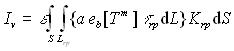(2)

wheredenotes integration along a line connecting r and p.

To carry out the surface integration arising in integrals Is and Iv the values of the kernel function K at a set of quadrature nodes should be known. This set of points is the same for both integrals. Additionally, both integrands contain a line integral. In the case of Is it is the transmissivity and in the case of Iv it is the integral in braces in Eq. 2. Both line integrals can be evaluated analytically. To do this, the medium is subdivided into cells and constant (but different) values of temperature and absorption coefficient is assumed within each cell. To carry out the integration three quantities should be known for each cell: the length of the ray within a given cell, the temperature of the cell and its absorption coefficient. All these quantities are calculated when discretizing the surface integral Is. Thus, all quantities needed to discretize the volume integral Iv are already calculated when processing the surface integral Is. The most time consuming procedure of the Hottel's zoning method, ie., the discretization of the volume integrals is performed in BEM almost for free.

Poor accuracy of numerical integration has been reported in the literature on zoning technique. Some authors propose to use regression or Lagrangian multipliers to correct the values of the calculated entries of the zoning matrices3. It has been shown2 that the reason of the error is the singular behaviour of the kernel functions K. The accuracy of numerical integration of such functions is poor because the polynomial interpolation used in quadratures cannot approximate singular variation of the integrand. The same problem has been encountered in standard BEM, where several techniques of handling this difficulty have been developed. The method used in the present work is based on a a priori error estimate of the Gaussian quadrature. This error is expressed in terms of the relative dis- tance of the surface element and the observation point4. It can be therefore checked, before the integration is carried out, whether the accuracy is below the prescribed threshold. If not, the surface element is subdivided into two subelements. The error of integration is then estimated for these subelements. If necessary, the subelements are further subdivided. The subdivision strategy is based on the octree algorithm used in computer graphics to store images. When using BEM, the conservation relations on the entries of the matrices can be satisfied with practically arbitrary accuracy, limited solely by the finite representation of numbers in the computer.

3. COUPLING OF HEAT RADIATION, CONVECTION AND CONDUCTION

Self irradiation of concave portions of boundaries makes the solution of the corresponding heat conduction problem difficult. In realistic problems, this portion of the boundary exchanges heat both by radiation and convection. The energy conservation equation for such boundary can be written as q = h(T - Tf) + qr where q- conductive heat flux, Tr- temperature of the radiating concave boundary, f- temperature in the core of the fluid, h- heat transfer coefficient. Radiative heat flux qr is a solution of an integral equation, the boundary condition at concave boundary is therefore non local. It depends on temperatures of all points of the boundary that can be seen from a given point.

Heat conduction problems in bodies containing radiative cavities require simultaneous solution of the differential equation of heat conduction and the integral equation of heat radiation. Steady state heat conduction in bodies consisting of several subregions of different conductivity are analyzed. The surface of the cavity is a gray and diffuse emitter and reflector of radiation. The medium filling cavities formed by concave boundaries is transparent to radiation. BEM is used in both conductive and radiative portions of the analysis.

BEM discretization of heat condition problem yields a set of algebraic equation having a form HT = Gq + Zqr . Where H, G and Z known matrices of constant coefficients.Vectors T, q and qr contain nodal values of boundary temperatures, conductive and radiative heat fluxes, respectively. Discretized equation of heat radiation has an appearance Aeb[Tr]+Bqr. A and B are known matrices of constant coefficients, vector eb stores nodal values of blackbody emissive powers at points placed on the radiating concave surface.

Equations of heat conduction and radiation are coupled by the presence of the radiative heat fluxes and temperatures at radiating boundaries. An efficient strategy of solving these sets has been developed. The first step is to eliminate the radiative heat fluxes from radiation and conduction equations using static condensation. The next step is the elimination of all unknowns except the temperatures of the radiating surfaces. The eliminations are carried out using a direct linear equations solver. The resulting nonlinear set of equations has the minimum possible number of unknowns. Newton Raphson solver proved to be a very efficient tool of solving the final set of equations. The technique has been extended to handle nonlinear material properties.

REFERENCES

1. Banerjee, P.K., Boundary Element Methods in Engineering, McGraw Hill, London, 1994
2. Bialecki, R.A., Solving Heat Radiation Problems Using the Boundary Element Method, Computational Mechanics Publications, Southampton and Boston, 1993.
3. Howell, J.R., Thermal radiation in participating media: the past, the present, and some possible futures. J. Heat Transf. Vol.110, No.4, pp 220-1229, 1988.
4. Bialecki, R.A., Dallner, R. and Kuhn, G., Minimum distance calculation between a source point and a boundary element, Eng. Anal. Bound. Elem., Vol. 12, No.12, pp 211-218, 1994.

1 Financial support of the Polish Committee of Scientific Research under grant T10B01411 is gratefully acknowledged herewith

APPLICATION OF NARROWBAND MODEL TO STUDY THE GAS PHASE RADIATIVE EFFECTS ON THE BURNING OF A SOLID FUEL

Hasan Bedir and James S. T'ien
Department of Mechanical and Aerospace Engineering
Case Western Reserve University, Cleveland, Ohio 44106, USA

ABSTRACT. Gas phase radiative effects on the burning of a solid fuel (PMMA) in a stagnation point flow geometry are investigated using a statistical narrowband model. Flame profiles calculated with and without the gas phase radiation are presented.

1. INTRODUCTION

Radiative heat transfer changes the temperature field in a flame and this modifies flame properties such as thickness and extinction boundaries and species production because of strong coupling between chemical kinetics and temperature field. Therefore accurate modeling of radiation is necessary . Narrowband models are considered to be the most accurate radiation treatment of nongray gases like carbondioxide and water vapor except for the line by line method. In this paper coupled system of mass, momentum, energy and radiative transport equations are solved numerically to show the effect of gas phase radiation on the burning of a solid fuel.

2. MATHEMATICAL MODEL

An axisymmetric stagnation point configuration is adopted for the solid fuel laminar diffusion flame analysis. The combustion model, with one step second order gas phase chemical reaction and a one step Arrhenius solid pyrolysis reaction, is similar to that described in [2, 3]. A radiative source term is added to the energy equation and the surface energy balance is modified to take into account the gas phase radiation.

The flame is assumed to be an infinite planar medium between two black walls, one at the solid fuel temperature and the other at ambient temperature. The thermodynamics properties are assumed to vary only with the direction perpendicular to the walls (y). Carbon dioxide, water vapor and fuel vapor are the only participating gases considered, soot radiation is neglected. Radiative source term in the energy equation is calculated from nongray radiative transport equation for an absorbing and emitting medium written in terms of mean transmittance over a narrowband . A statistical narrowband model with an exponential tailed inverse line strength distribution  is used to calculate transmittance of CO2 and H2O. Mean band parameters data, generated by Soufiani and Taine from their line by line calculations  using HITRAN 92 database, are employed. Statistical narrowband model parameters of MMA vapor are taken from . Transmittance of nonisothermal and inhomogeneous medium is approximated by the transmittance of an equivalent isothermal and homogeneous medium (Curtis-Godson approx.). The radiative transport equation is discretized according to SN discrete ordinates method (20 direction Gaussian quadrature) and solved numerically to obtain radiative heat flux and its divergence. These values are used in the combustion model to calculate the temperature and species profiles which are coupled back to the radiative transport equation. This iterative procedure is repeated until the solution is converged.Figure l: Effect of gas phase radiation on temperature and species partial pressures. PMMA burning in air, stretch rate is 10 s-1.Figure 2: Spectral heat flux to the fuel surface and ambient.

3. RESULTS

Fig. 1 shows the effect of gas phase radiation on the burning of PMMA in air for the case with stretch rate (velocity gradient of the air jet) of 10 s-l. When the gas phase radiation is included the maximum flame temperature is lower (7%). The reaction zone where oxidizer and fuel meet and reacts moves closer to the fuel surface, hence there are less products at the ambient side of the flame. Fuel burning rate, determined through a surface pyrolysis law, is a function of the net heat flux at the fuel surface. Since the flame temperature is lower, conduction heat flux to the surface is decreased when the gas phase radiation is included. However the radiation from the flame to the surface makes up for this decrease and the burning rate is 4% larger for this particular flame. The spectral radiation heat fluxes are shown in Fig. 2. Surface radiative heat loss (qw+) and ambient radiation (qe-) are blackbody radiations at corresponding wall and ambient temperatures. The contributions of various gas bands to the flame radiation to the surface (qw-) and flame radiation to ambient (qe+) can be seen from the flgure. The largest contribution is by the strong 4.3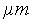CO2 band followed by MMA vapor bands.

The authors acknowledge the support by NASA Microgravity Science and Applications Division grant NAG3-1046

4. REFERENCES

1. Rhatigan, J.L., Bedir, H., T'ien, J.S., Gas phase radiative effects on the burning and extinction of a solid fuel, Combust. and Flame, in press.
2. T'ien, J.S., Combust. and Flame, Vol.65, pp 31-34,1986.
3. T'ien, J.S. et al, Combust. and Flame, Vol.33, pp 55-68, 1978.
4. Kim, T.K., Menart, J.A., and Lee, H.S., J. Heat Transf., Vol.ll3, pp 946-951, 1991.
5. Malkmus, W., J. Opt. Soc. Am., Vol.57, pp 323-329, 1967
6. Soufiani, A. and Taine, J., Int. J. of Heat and Mass Transf., Vo1.40, p 987,1997.
7. Park, S.H., Stretton, A.J., Tien, C.L., Combust. and Flame, Vol.62, pp 257-271, 1988.

NUMERICAL SIMULATION OF RADIATIVE-CONVECTIVE INTERACTION IN NOZZLES OF LASER PLASMA ACCELERATORS

S. T. Surzhikov
Institute for Problems in Mechanics Russian Academy of Sciences, Moscow, Russia

Laser Plasma Accelerator (LPA) is defined as a Laval nozzle, inside which gas is heated up by means of laser radiation (Fig.l).Fig.l Scheme ot the Laser Plasma Accelerator

In the study a radiative mode of LPA is investigated. Distinctive feature of the radiative mode of LPA is the fact that cold gas overfilling on laser plasma is heated up to temperature of unitary ionization by means of own thermal radiation of laser plasma.

The 2D radiative gas-dynamic model of LPA is developed, in which selective radiating heat exchange of laser plasma with environment is taken into account. The governing equation of the gas flow in the plane Laval nozzle are the Navier-Stokes equations, mass and energy conservation equations, heat radiative and laser radiative transfer eduations:are the density, specific internal energy, pressure, velocity components along x and y axes, respectively; Wx,g, Wy,g are the group heat radiative flux eomponents along x and y axes; Ug is the group density of heat radiative energy,are the spectral and group radiative intensities; index b denotes characteristics of a black body;is the direction of radiation propagation,is the element of solid angle; kg is the group absorption coefficient,is the laser radiation absorption coefficient;are the heat conductivity coefficient and dynamic viscosity coefficient;is the specific heat at constant volume; eo is the specific internal energy at the temperature To; yL(x) is conventional boundary of laser beam. The group functions (fg = kg, Ug, Wx,g, Wy,g) are obtained by the following integration of the corresponding spectral functions (fw = kw, Uw, Wx,w, Wy,w):, whereis the g-th wavenumber scale range. The total radiative heat flux is obtained by the following summation:, where Ng is the number of spectral groups.

The multigroup model of the group absorption coefficient kg is calculated at the atmospheric pressure in the temperature region 300-20000 K with the temperature stepT= 200 K. The 18 spectral groups are taken into account in the spectral region 2000-150000 1/cm. The thermophysical properties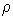(T),(T) , and equilibrium composition of heated air are calculated at the same temperature points.

The numerical scheme used to calculate the steady-state solution via a pseudotransient formulation is based on a second order finite difference discretization of the flux gradients and a separate time-integration method. The full implicit schemes are used for solution of all equations. The typical results of the calculations are shown on Fig.2.

It is necessary to stress that the problem under consideration is the strongest self-consistent problem when temperature and gas dynamic distributions are developed each from other. And the radiative heat transfer processes are of primary importance in determining the gas dynamically fields inside the Laval nozzles of the LPA.Fig.2 The isotherms (dashed lines) in the curvilinear coordinate system and distribution of the temperature along the x-axis (line with crosses) in the symmetry plane (the scale on the right, the temperature in kK) in a subsonic Laval nozzle at PL=30 kW/cm and uo=3 m/sec. The laser radiation and gas flow are directed from left to right

MODELING OF HEAT AND MOISTURE TRANSFER IN THIN CAPILLARY-POROUS MATERIALS UNDER HIGH INTENSITY HEATING BY RADIATION

R.Sh. Enalejev, W.A. Katchalkin
Department of Cybernetics, Kazan Chemical Technological University, Russia

INTRODUCTION

Investigation of heat and moisture transfer into thin capillary-porous materials under high intensity heating by radiation has both theoretical and practical value in solving of many problems in such spheres as heat protection of aerocosmic construction , intensification of drying processes , determination of high intensity heating mechanism .

However, mechanism of interior mass and energy transfer into thin materials accompanied by phase transformation is still insufficiently known, because it is difficult to measure the field of potential transfer.

Mathematical description of transfer process circumscribe the system of nonlinear differential equations of parabolic type. Complicacy of equations and absence of sufficient data on process characteristics causes insuperable mathematical difficulties during the solution of compatible heat and mass transfer equations.

That is why we suggest the simplified mathematical models for investigation of interaction processes of high intensity radiation with thin capillary-porous materials. Construction of models is based on the mathematical simulation method.

HEAT AND MASS TRANSFER MODEL

The results of mathematical modeling of heat and moisture transfer process in wet textile materials under pulsed radiant heating are presented in this paper. Adequacy of mathematical model to experimental data was determined according to parameters which characterize joint heat and moisture transfer: temperature of sample, loss of sample mass due to radiation, heat balance of heating process of material and moisture evaporation.Figure1. Model of heat and moisture transfer

As it is shown on figure 1, the process of vigorous vaporization of moisture on the whole body of the sample begins just from the very start of radiation process. The process of ebullition of moisture begins when the temperature on the surface is about 85°C. Under further heating the ebullition border moves deep down the material, the rate of deepening depends on intensity of radiation, moisture - content, thermophysical and optical properties of material. Ebullition border divides material into dry and wet zones. Depending on heating intensity temperature of deep layers behind the mobile border may mount to the temperature of ebullition, and this causes the advent of the second mobile border.

Ebullition zone is observed between the two mobile borders; due to vigorous vaporization an excess pressure arises here. Under the influence of pressure gradient molar flows of vapour and free water are formed. The movement of free water in high temperature zone is accompanied by its further evaporation.

Preliminary calculations have shown that diffusion flows of heat and moisture are negligible.

MATHEMATICAL FORMULATION

To determine the temperature fields and moisture content it is necessary to solve the system of nonlinear differential equations of heat conductivity with volumetrical source of heat, mobile borders of phase transition and equation of moisture conductivity.

Absence of data on pressure gradient excludes the possibility of solving the equation of moisture conductivity in its classical formulation .

That is why we suggest expressing the rate of evaporation in the equation of moisture conductivity through the part of absorbed radiation energy spent on evaporation of moisture. It is possible to realize such an approach by introducing of differential Cossovitch criterion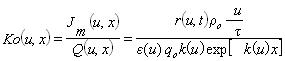(1)

which (for differential volume) characterizes the ration of heat spent on evaporation -Jm(u,x) to heat absorbed by wet material due to radiation Q(u,x).

Then we can present the equation of energy mathematical formulation in the following way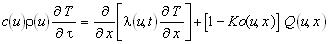(2)

CONCLUSIONS

• The mathematical model adequately describing the process of heat and moisture transfer in thin capillary-porous materials under the influence of intense light radiation is presented.
• The main mechanisms of inner heat and moisture transfer are determined by mathematical modeling method and their quantitative estimation is also carried out.
• The usage of high temperature sources of radiation can increase the rate of drying tens times as much in comparison with other ways of intense heating

REFERENCES

1. Al. Kuryachii . Evalution of system efficency of radiation-evaporating heat protection of flying apparatus. // Mechanics of liquids and gases, 1995, pp. 24-36.
2. Chen and D. Pei. A mathematical model of drying processes. // Int. J. Heat Mass Transfer. Vol. 32. ? 2 ,1989,pp 297-310.
3. M.D.Mikhailov. Exact solution of temperature and moisture distribution in a porous half-space with moving evaporation front, Int. J. Heat Mass Transfer 18,1975, pp 797-804 .
4. A.V.Luikov. Systems of differential equations of heat and mass transfer in capillary-porous bodies. Int. J. Heat Mass Transfer 18, 1975, pp 1-14.

NUMERICAL SIMULATION OF THERMAL STRESSES TAKING PLACE IN SEMITRANSPARENT MATERIALS UNDER LASER RADIATION.

Katasonov A.A., Kokurina G.P., Rumynsky A.N.,

One of the reasons of materials destruction under laser radiation is occurrence of thermal stresses due to non-uniform material heating. Investigations of thermal stresses in opaque and transparent materials were carried out in a lot of works, this being not so for semitransparent materials because in this case it is necessary to take into account additionally the process of radiation/conduction heat transfer in scattering media.

In this work ìn non-connected thermal elasticity approximation we consider thermal stresses induced by continuos laser radiation in semitransparent materials with due regard for consumption and scattering of both falling radiation and that from heated material.

We consider a cylinder of elastic-isotropic uniform material. Axisymmetrical radiation flux with Gaussian intensity radius distribution, reaching its stationary regime at some characteristic time to falls on its face surface along the normal.

The complete set of equations describing thermal elastic effect of laser radiation upon semitransparent material includes equations of radiation/conduction energy transfer (energy transfer equation and parabolic heat conduction equation with a source member) and equations of thermoelasticity.

We suppose that the radiation energy transfer equation is valid for this media, semitransparent material is a grey media with transparent boundaries, and all materials characteristics are temperature independent. The consideration of radiation transfer may be divided into two independent problems:

• radiation transfer due to falling laser one;
• radiation transfer due to that from heated material.

The calculation of radiation transfer due to falling laser one should be carried out in the most thorough way because of its mono-direct character, so the radiation energy transfer equation in exact formulation should be used. We write this equation in the integral form and use Monte-Carlo method local estimators with Calos' modification) for the calculation of radíation consumption for the established regime of laser beam.

The diffusion approximation of radiation energy transfer equation may be used for the calculation of radiation transfer due to that from heated material for every time moment.

The appearance of other equations is obvious.

The solution of the whole problem may be divided into following independent stages:

• the consumption of radiation due to falling laser one is calculated once for the established regime;
• the calculation of radiation/conduction heat transfer for selected time period;
• the calculation of thermal stresses for chosen time moments.

It is very convenient to use the finite elements method for the solution of equations of heat conduction and thermoelasticity and for the calculation of absorption of radiation from heated material.

The calculations were carned out for specimens of hypothetical ceramics with both radius R and thickness L equal to 1 cm, energy flux in the beam centre Fo=1.5 kWt/cm2, beam effective radius ro=0.2 cm. At calculations we varied specimen optical lengthand single-scattering albedo qe .

Analysis of calculations results shows that thermal stresses distribution significantly depends on optical parameters of sernitransparent materials. First of all thìs effect manifests itself in behaviour of axial component of stress tensorup to change of sign.

BASE RADIATION HEAT TRANSFER DURING OPERATION OF ROCKET ENGINES

Rumynsky A.N., Katasonov A.A., Osipov V.A., Pugatchev V.A.

The problem of vehicle base heating due to thermal radiation of exhaust gaseous and two-phase jets (solid fuel engines) is known to be important for design of rockets heat protection. Determination of radiation from such jets is rather difficult and includes some separate problems:

• calculation of exhaust gaseous or two-phase jets from nozzle exit and determination of its parameters (pressure, temperature, chemical composition of gas mixture, thermal and phase state of condensed particles);
• calculation of gas emission and optical properties of particles (absorptíon and scattering coeff cients and phase function);
• determination of radiation heat lux to the vehicle elements.

Each of these problems enumerated in turns requires the preliminary decision of a number of questions. For example, the calculation of two-phase flow in nozzle must be carried out before determination of parameters in exhaust jet. Furthermore the thermodynamic calculation in combustion chamber should be obtained.

The calculation of thermal radiation from some gaseous jets for multi-nozzle engine is a separate problem. The interaction of supersonic jets will take place during vehicle operation. This interaction leads to appearance of the shock waves and, as a result, to the existence of regions in which gas will have high temperature and pressure. These regions are main sources of thermal radiation to the vehicle elements.

The problems mentioned above are solved using a number of simplifications which have to meet the following demands:

• the method must allow to calculate radiation flux with acceptable accuracy (for engineer applications);
• the program must allow to carry out calculations at the acceptable time.

Because of these demands some questions are artificially simplified. For example, non-equilibrium chemical reactions in gas phase are excluded, particles are considered to be monodisperse, wave interaction between gas jets is calculated using oblique shock formulae, etc.

The programs for determination of radiation characteristics from gaseous or two-phase jet use three large modules:

1. "INIT" - calculation of equilibrium gas mixture in nozzle and so, determination the initial condition for next modules;
2. "JET" - calculation of two/single - phase flow in axisymmetrical jet flow parameters, extended into flooding flow (Godunov's method is used);
3. "RAD" - determination of radiation heat flux to arbitrary point of vehicle base (Ruminsky's undetermined coefficients method is used for the calculation of radiation transfer in scattering media, Reardon's line model for the gas emittance and Mie' theory for particles emittance are used.

The comparison of the results of these calculations with available experimental data shows good agreement.